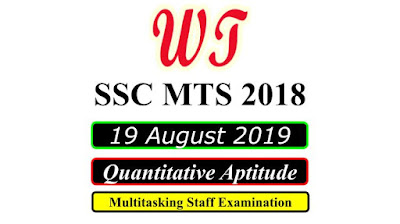Type Here to Get Search Results !

# [Download] SSC MTS 19 August 2019, Quantitative Questions PDF

Download SSC (Staff Selection Commission) MTS - Multi Tasking (Non-Technical) Staff Examination 2018 Tier 1 All Quantitative Aptitude Questions , Was Held on 19 August 2019 All Quantitative Questions PDF With Answers.

Table of Content

Table of Content (toc)

## SSC MTS 19 August 2019 All Shifts Quantitative Questions PDF Download FreeSSC MTS 19 August 2019 All Shifts Quantitative Questions PDF Download Free

### SSC MTS 19 August 2019 Shift 1 Quantitative Questions

Instructions
For the following questions answer them individually
Q1:-Pipe A can fill a tank in 10 hours. Pipe B can fill the same tank in 12 hours. Pipe C can empty the full tank in 16 hours. All the pipes are opened at 8 : 00 A.M. and Pipe A and B are closed at 10 : 00 A.M. After how much time from starting will the tank be empty?
a.    5 hours 52 minutes
b.    5 hours 24 minutes
c.    4 hours 30 minutes
d.    4 hours 8 minutes
Q3;-Cost price of an article is ₹1440 and its selling price is ₹1800. What is the profit percentage?
a.    15%
b.    25%
c.    20%
d.    12.5%
Q4:-What is the greatest number which can exactly divide 192, 1056 and 1584?
a.    48
b.    56
c.    44
d.    36
Q5:-The sum of two numbers x and is 48 and their difference is 6. Then x : y = ?
a.    8 : 9
b.    7 : 6
c.    9 : 7
d.    3 : 7
Q6:-A sum is divided between two persons in the ratio 3 : 2. If one person got ₹12 less than the other person, then what is the sum?
a.    ₹60
b.    ₹50
c.    ₹72
d.    ₹44
Q7:-What is the compound interest on ₹5000 in 2 years at the rate of 20% per annum?(interest compounded half yearly)
a.    ₹2340.50
b.    ₹2275.50
c.    ₹2290.50
d.    ₹2320.50

### SSC MTS 19 August 2019 Shift 2 Quantitative Questions

Q2:-An amount is divided between Ram and Rabin in the ratio of 3 : 2. If Ram’s share is ₹36000, then what is the total amount?
a.    ₹60000
b.    ₹72000
c.    ₹90000
d.    ₹40000
Q4:-A boat can tavel 10 km upstream and 20 km downstream in 7 hours. It can travel 20 km upstream and 10 km downstream in 11 hours. What is the speed of bout in still water?
a.    4 km/h
b.    2 km/h
c.    6 km/h
d.    8 km/h
Q5:-The ratio between two given numbers is 9 : 7. If each number is increased by 6, then the ratio becomes 21 : 17. What is the difference Between a the now given numbers?
a.    16
b.    8
c.    4
d.    12
Q9:-The average weight of 13 students and their teacher is 24.5 kg. To the weight of the teacher is 31 kg. then what is the average weight of the 13 students?
a.    23.5 kg
b.    25 kg
c.    24 kg
d.    23 kg
Q10:-What is the difference between the mean and the median of the following data: 5, 7, 8, 13, 12, 14, 9, 2, 26, 10 ?
a.    2.3
b.    0.4
c.    1.8
d.    1.1
Q12:-Speed of a car is 20 m/s. How much distance (in km) will the car travel in 2.5 hours?
a.    180
b.    108
c.    72
d.    144

### SSC MTS 19 August 2019 Shift 3 Quantitative Questions

Instructions
For the following questions answer them individually
Q1:-A trader sells two articles for ₹9520 each, one at a profit of 12% and the other at a loss of 15%. His overall percentage loss (correct to one decimal place) is:
a.    4.4
b.    3.1
c.    3.4
d.    3.8
Q2:-A person travels a certain distance at a speed of 18 km/h and returns to the starting point at 12 km/h. If he takes 2 hours 55 minutes for the whole journey, the distance one way is:
a.    21 km
b.    25 km
c.    18 km
d.    24 km
Q4:-What will be the compound interest on a sum of ₹31250, at 12% compound interest (interest compounded yearly) in years?
a.    ₹11048
b.    ₹11096
c.    ₹11068
d.    ₹11086
Q5:-Let x be the smallest number greater than 600 which gives the remainders 2, 3 and 4, when divided by 5, 6 and 7, respectively. The sum of digits of x is:
a.    14
b.    15
c.    13
d.    16
Q7:-An item is sold for ₹702 at a profit of ₹162. If an additional! profit of 10% is to be made, then the new selling price will be:
a.    ₹756
b.    ₹729
c.    ₹810
d.    ₹750
Q8:-In an examination, the average score of students in a class who passed is 69 and that of who failed is 61. If the average score of all the students, who appeared in the examination is 66, then the percentage of students who passed is:
a.    37.5
b.    60
c.    62.5
d.    56

SSC MTS Previous Year Paper

SSC MTS 2018

SSC MTS Reasoning Questions

SSC MTS Quantitative Questions

SSC MTS General English Questions

SSC MTS General Knowledge